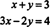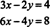## Solutions to Linear Systems

The analysis of linear systems will begin by determining the possibilities for the solutions. Despite the fact that the system can contain any number of equations, each of which can involve any number of unknowns, the result that describes the possible number of solutions to a linear system is simple and definitive. The fundamental ideas will be illustrated in the following examples.

Example 1: Interpret the following system graphically:Each of these equations specifies a line in the x−y plane, and every point on each line represents a solution to its equation. Therefore, the point where the lines cross—(2, 1)—satisfies both equations simultaneously; this is the solution to the system. See Figure .Figure 1

Example 2: Interpret this system graphically:The lines specified by these equations are parallel and do not intersect, as shown in Figure . Since there is no point of intersection, there is no solution to this system. (Clearly, the sum of two numbers cannot be both 3 and −2.) A system which has no solutions—such as this one—is said to be inconsistent.Figure 2

Example 3: Interpret the following system graphically:Since the second equation is merely a constant multiple of the first, the lines specified by these equations are identical, as shown in Figure . Clearly then, every solution to the first equation is automatically a solution to the second as well, so this system has infinitely many solutions.Figure 3

Example 4: Discuss the following system graphically:Each of these equations specifies a plane in R 3. Two such planes either coincide, intersect in a line, or are distinct and parallel. Therefore, a system of two equations in three unknowns has either no solutions or infinitely many. For this particular system, the planes do not coincide, as can be seen, for example, by noting that the first plane passes through the origin while the second does not. These planes are not parallel, since v 1 = (1, −2, 1) is normal to the first and v 2 = (2, 1, −3) is normal to the second, and neither of these vectors is a scalar multiple of the other. Therefore, these planes intersect in a line, and the system has infinitely many solutions.

Example 5: Interpret the following system graphically:Each of these equations specifies a line in the x−y plane, as sketched in Figure . Note that while any two of these lines have a point of intersection, there is no point common to all three lines. This system is inconsistent.Figure 4

These examples ilustrate the three possibilities for the solutions to a linear system:

Theorem A. Regardless of its size or the number of unknowns its equations contain, a linear system will have either no solutions, exactly one solution, or infinitely many solutions.

Example 4 illustrated the following additional fact about the solutions to a linear system:

Theorem B. If there are fewer equations than unknowns, then the system will have either no solutions or infinitely many.